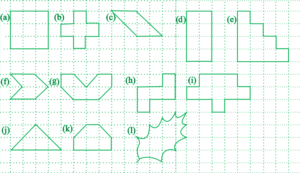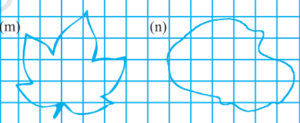# Free Ncert Class 6 Math Mensuration Exercise 10.2

Mensuration Class 6 Ex. 10.2

Mensuration, Ncert Class 6, Chapter 10, Exercise 10.2 Math Free Solution.

Exercise 10.2

Question 1 :- Find the areas of the following figures by counting square:Solution 1 :-
(a) Number of full squares = 9
Area of 1 square = 1 square unit;
Since, the area of 9 squares = 9 x 1 square unit = 9 square units;
So, the area of the portion covered by 9 squares = 9 square units.

(b) Number of full squares = 5
Area of 1 square = 1 square unit;
Since, the area of 5 squares = 5 x 1 square unit = 5 square units;
So, the area of the portion covered by 5 squares = 5 square units.

(c) Number of full squares = 2
Number of half squares = 4
Since, the area of the covered figure = 2 x 1 + 4 x (1/2) = 2 + 2 = 4 square units.

So, the area of the portion covered = 4 square units.

(d) Number of full squares = 8
Area of 1 square = 1 square unit;
Since, the area of 8 squares = 8 x 1 square unit = 8 square units;
So, the area of the portion covered by 8 squares = 8 square units.

(e) Number of full squares = 10
Area of 1 square = 1 square unit;
Since, the area of 10 squares = 10 x 1 square unit = 10 square units;
So, the area of the portion covered by 10 squares = 10 square units.

(f) Number of full squares = 2
Number of half squares = 4
Since, the area of the covered figure = 2 x 1 + 4 x (1/2) = 2 + 2 = 4 square units.

So, the area of the portion covered = 4 square units.

(g) Number of full squares = 4
Number of half squares = 4
Since, the area of the covered figure = 4 x 1 + 4 x (1/2) = 4 + 2 = 6 square units.

So, the area of the portion covered = 6 square units.

(h) Number of full squares = 5
Area of 1 square = 1 square unit;
Since, the area of 5 squares = 5 x 1 square unit = 5 square units;
So, the area of the portion covered by 5 squares = 5 square units.

(i) Number of full squares = 9
Area of 1 square = 1 square unit;
Since, the area of 9 squares = 9 x 1 square unit = 9 square units;
So, the area of the portion covered by 9 squares = 9 square units.

(j) Number of full squares = 2
Number of half squares = 4
Since, the area of the covered figure = 2 x 1 + 4 x (1/2) = 2 + 2 = 4 square units.

So, the area of the portion covered = 4 square units.

(k) Number of full squares = 4
Number of half squares = 2
Since, the area of the covered figure = 4 x 1 + 2 x (1/2) = 4 + 1 = 5 square units.

So, the area of the portion covered = 5 square units.

(l) Number of full squares = 4
Number of squares more than half = 3
Number of half squares = 2
So, the area of the covered figure = [4 x 1 + 3 x 1 + 2 x (1/2)] square units
= (4 + 3 + 1) square units = 8 square units.

(m) Number of full squares = 6
Number of squares more than half = 8
So, the area of the covered figure = [6 x 1 + 8 x 1 ] square units
= (6 + 8) square units = 14 square units.

(n) Number of full squares = 9
Number of squares more than half = 9
So, the area of the covered figure = [9x 1 + 9 x 1 ] square units
= (9 + 9) square units = 18 square units.